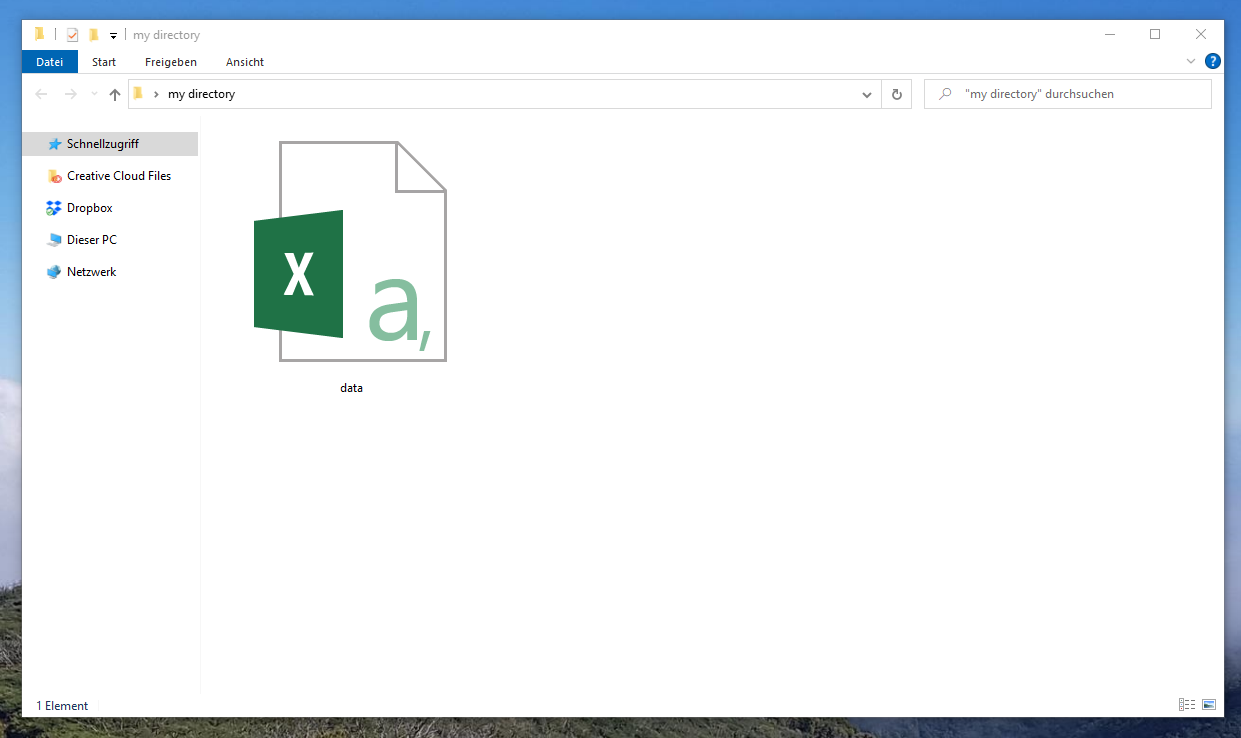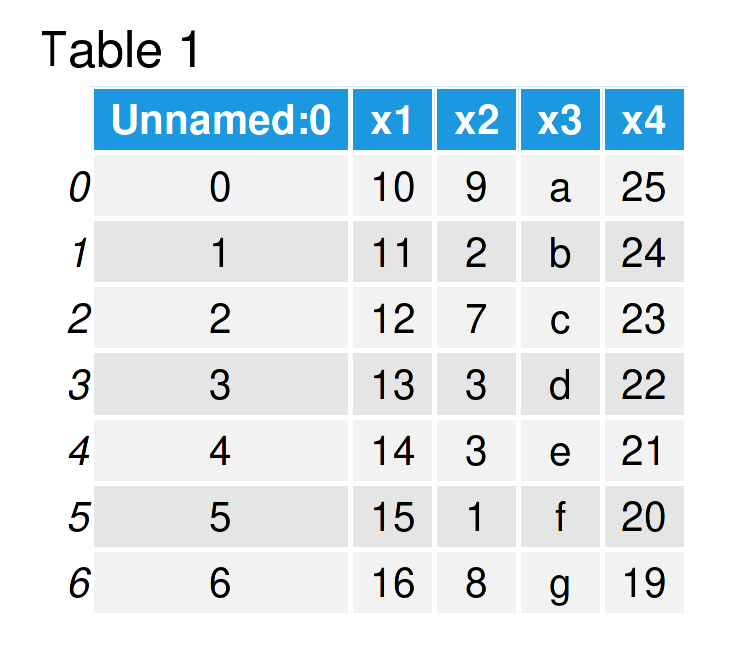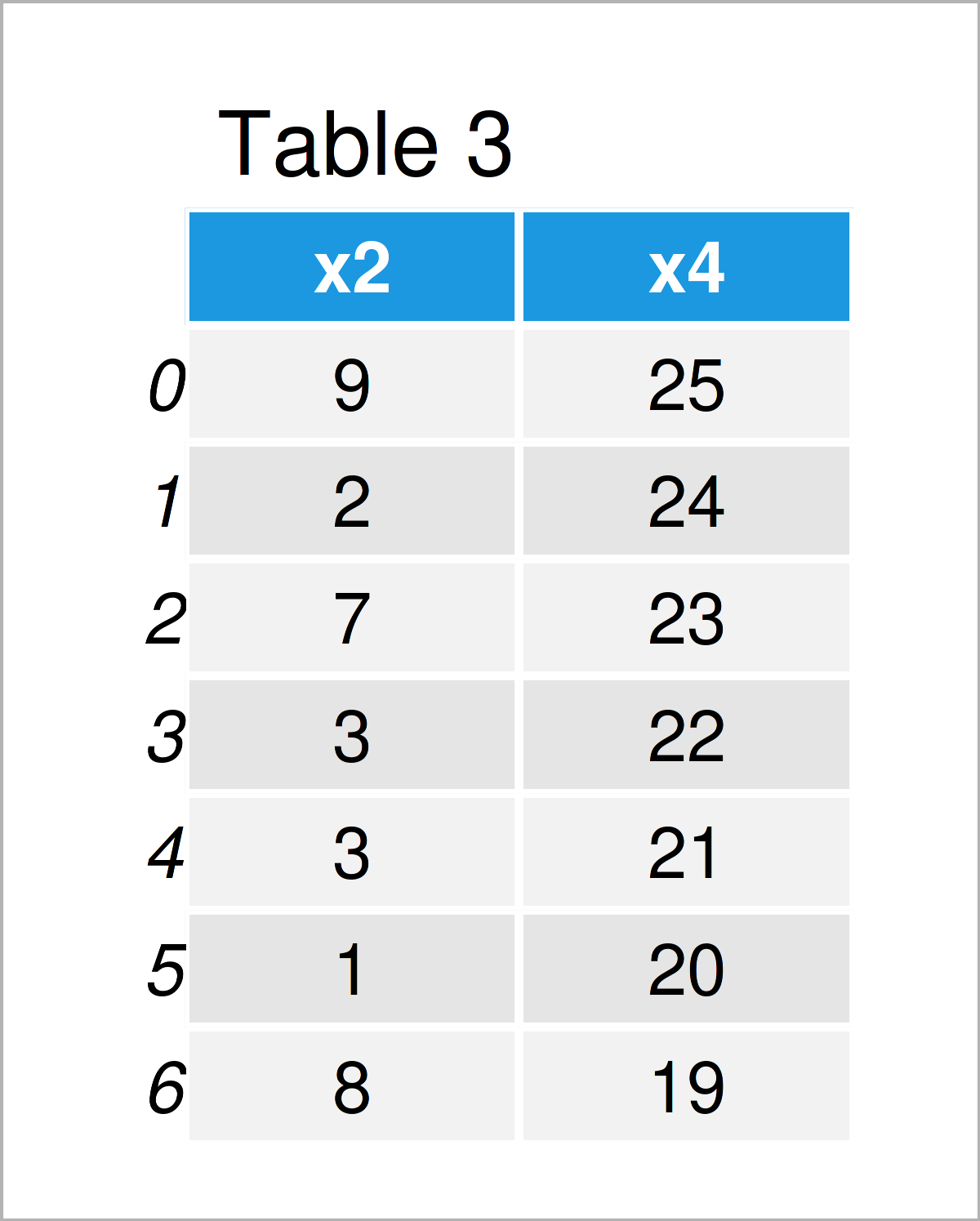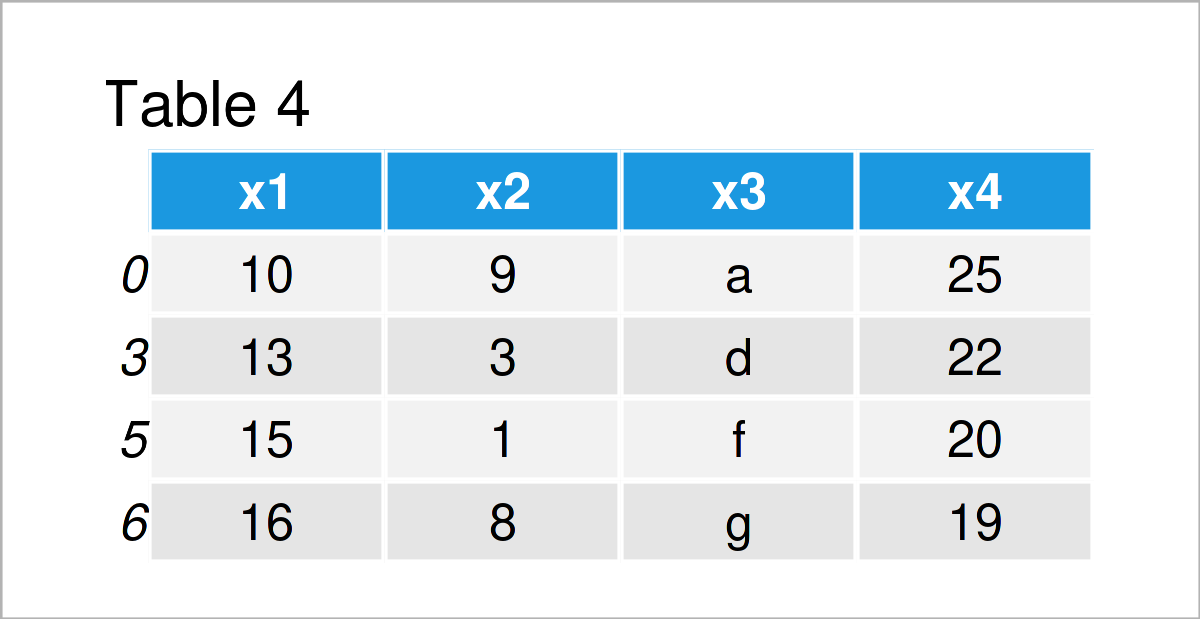# Read CSV File as pandas DataFrame in Python (5 Examples)

In this article, I’ll demonstrate how to import a CSV file as a pandas DataFrame in the Python programming language.

Let’s just jump right in!

## Example Data & Libraries

We first have to import the pandas library:

`import pandas as pd                                # Import pandas library to Python`

Next, we’ll have to create an exemplifying CSV file for the examples of this tutorial. For this, we’ll use the pandas DataFrame below:

```data = pd.DataFrame({'x1':range(10, 17),           # Create pandas DataFrame
'x2':[9, 2, 7, 3, 3, 1, 8],
'x3':['a', 'b', 'c', 'd', 'e', 'f', 'g'],
'x4':range(25, 18, - 1)})```

If we want to save this pandas DataFrame as a CSV file on our computer, we also have to specify the location where we want to store it.

To do this, we need to load the os module to Python:

`import os                                          # Load os module`

Now, we can use the chdir function to specify the path to a folder that we want to use in this example:

`os.chdir('C:/Users/Joach/Desktop/my directory')    # Set working directory`

And finally, we can export our example data set as a CSV file to this folder using the to_csv function:

`data.to_csv('data.csv')                            # Export pandas DataFrame`

After the execution of the previous Python codes, our working directory looks like this:As you can see, our working directory contains a single CSV file. This file contains the pandas DataFrame that we have created above.

In the following examples, I’ll show different ways on how to load these data as a pandas DataFrame into Python.

Let’s do this!

## Example 1: Import CSV File as pandas DataFrame Using read_csv() Function

In Example 1, I’ll demonstrate how to read a CSV file as a pandas DataFrame to Python using the default settings of the read_csv function.

Consider the Python syntax below:

```data_import1 = pd.read_csv('data.csv')             # Read pandas DataFrame from CSV
print(data_import1)                                # Print imported pandas DataFrame```Table 1 shows the structure of the pandas DataFrame that we have just created: It consists of seven rows and five columns.

As you can see in the previous Python code, we did not have to specify the path to the working directory where the CSV file is located. The reason for this is that we have set the current working directory already in the previous section (i.e. Example Data & Libraries).

In case you have not specified the working directory yet, you would either have to do that using the chdir function as explained in the previous section; Or you would have to specify the path to the working directory in front of the file name within the read_csv function (i.e. pd.read_csv(‘C:/Users/Joach/Desktop/my directory/data.csv’)).

Note that the first column of the output DataFrame above contains index values. In the next example, I’ll show how to avoid that, so keep on reading!

## Example 2: Read CSV File without Unnamed Index Column

In Example 2, I’ll demonstrate how to load a CSV file as a pandas DataFrame with no Unnamed index column.

To accomplish this, we have to set the index_col argument to be equal to  as shown in the following Python syntax:

```data_import2 = pd.read_csv('data.csv',             # Read pandas DataFrame from CSV
index_col = )
print(data_import2)                                # Print imported pandas DataFrame```As shown in Table 2, we have created another pandas DataFrame that does not contain the Unnamed index column (as in Example 1).

## Example 3: Load Only Particular Columns from CSV File

It is also possible to create a pandas DataFrame that contains only some of the variables from a CSV file.

The following Python programming code explains how to do that based on our example file.

For this, we have to assign a list of column names to the usecols argument within the read_csv function.

Have a look at the example code below:

```data_import3 = pd.read_csv('data.csv',             # Read pandas DataFrame from CSV
usecols = ['x2', 'x4'])
print(data_import3)                                # Print imported pandas DataFrame```Table 3 shows the output of the previous Python syntax: A pandas DataFrame that consists only of the variables x2 and x4.

## Example 4: Skip Certain Rows when Reading CSV File

In Example 3, I have illustrated how to ignore certain columns when importing a data set from a CSV file. This example, in contrast, demonstrates how to avoid particular rows of a CSV file.

For this task, we can use the skiprows argument as shown below. As you can see, we have to assign a list of row numbers that we do not want to read to this argument.

Note that we are simultaneously using the index_col argument (as explained in Example 2) to exclude the indices from our CSV file.

```data_import4 = pd.read_csv('data.csv',             # Read pandas DataFrame from CSV
index_col = ,
skiprows = [2, 3, 5])
print(data_import4)                                # Print imported pandas DataFrame```As shown in Table 4, we have created a pandas DataFrame where some lines of the CSV file have not been imported. This can be especially useful when we are dealing with large data sets.

## Example 5: Set New Column Names when Reading CSV File

Another thing we can do when we open a CSV file is that we modify the names of the variables in this file.

This section explains how to change the column names of a CSV file during the reading process.

For this task, we have to assign a list of character strings that we want to set as new column names to the names argument of the read_csv function.

Furthermore, it makes sense to skip the first row of our input data, since this row contains the original header of our CSV file.

Let’s do this in practice:

```data_import5 = pd.read_csv('data.csv',             # Read pandas DataFrame from CSV
index_col = ,
skiprows = 1,
names = ['col1', 'col2', 'col3', 'col4'])
print(data_import5)                                # Print imported pandas DataFrame```Table 5 shows the output of the previous Python programming code – We have loaded our CSV file as a pandas DataFrame where we have converted the column names to new names.

## Video & Further Resources

Would you like to learn more about the importing and parsing of a CSV file as a pandas DataFrame? Then I can recommend having a look at the following video on my YouTube channel. In the video, I’m explaining the content of this post in Python.

In addition, you could read the other articles on www.statisticsglobe.com. Some articles on handling CSV files and other related topics are listed below.

On this page you have learned how to read and parse a CSV file as a pandas DataFrame in the Python programming language. Let me know in the comments section, in case you have any further questions.

Subscribe to the Statistics Globe Newsletter